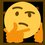# Equation of Simple Harmonic Motion of Spring Derivation

Hooke's Law states that:

\begin{aligned} F&=-kx\\ F+kx&=0\\ \frac{F}{m}+\frac{kx}{m}&=0\\ a+\frac{kx}{m}&=0\\ \frac{d^2x(t)}{dt^2}+\frac{kx(t)}{m}&=0\\ \end{aligned}

Assume $x(t)\propto e^{\lambda t}$ solves the differential equation:

\begin{aligned} \frac{d^2e^{\lambda t}}{dt^2}+\frac{ke^{\lambda t}}{m}&=0\\ \lambda^2e^{\lambda t}+\frac{ke^{\lambda t}}{m}&=0\\ e^{\lambda t}\left(\lambda^2+\frac{k}{m}\right)&=0\\ \lambda^2+\frac{k}{m}&=0\\ \lambda^2&=-\frac{k}{m}\\ \lambda&=\pm i\sqrt{\frac{k}{m}} \end{aligned}

$\begin{cases} x_1(t)=c_1e^{i\sqrt{\frac{k}{m}} t}\\ x_2(t)=c_2e^{-i\sqrt{\frac{k}{m}} t} \end{cases}$

\begin{aligned} x(t)&=x_1(t)+x_2(t)\\ &=c_1e^{i\sqrt{\frac{k}{m}} t}+c_2e^{-i\sqrt{\frac{k}{m}} t}\\ &=c_1\left[\cos\left(\sqrt{\frac{k}{m}}t\right)+i\sin\left(\sqrt{\frac{k}{m}}t\right)\right]+c_2\left[\cos\left(\sqrt{\frac{k}{m}}t\right)-i\sin\left(\sqrt{\frac{k}{m}}t\right)\right]\\ &=c_1\cos\left(\sqrt{\frac{k}{m}}t\right)+c_1i\sin\left(\sqrt{\frac{k}{m}}t\right)+c_2\cos\left(\sqrt{\frac{k}{m}}t\right)-c_2i\sin\left(\sqrt{\frac{k}{m}}t\right)\\ &=(c_1+c_2)\cos\left(\sqrt{\frac{k}{m}}t\right)+(c_1-c_2)i\sin\left(\sqrt{\frac{k}{m}}t\right)\\ &=C_1\cos\left(\sqrt{\frac{k}{m}}t\right)+C_2\sin\left(\sqrt{\frac{k}{m}}t\right)\\ &=\sqrt{C_1+C_2}\cos\left[\sqrt{\frac{k}{m}}t-\tan^{-1}\left(\frac{C_2}{C_1}\right)\right]\\ \end{aligned}

Let $A=\sqrt{C_1+C_2}$, $\omega^2=\frac{k}{m}$ and $\tan(\varphi)=\frac{C_2}{C_1}$:

$\boxed{x(t)=A\cos\left(\omega t-\varphi\right)}$

Proof by differentiation:

\begin{aligned} x(t)&=A\cos\left(\omega t-\varphi\right)\\ \frac{dx(t)}{dt}&=-A\omega\sin\left(\omega t-\varphi\right)\\ \frac{d^2x(t)}{dt^2}&=-A\omega^2\cos\left(\omega t-\varphi\right)\\ \frac{d^2x(t)}{dt^2}&=-\omega^2[A\cos\left(\omega t-\varphi\right)]\\ \frac{d^2x(t)}{dt^2}&=-\frac{k}{m}x(t)\\ \frac{d^2x(t)}{dt^2}+\frac{kx(t)}{m}&=0\quad\blacksquare \end{aligned}Note by Gandoff Tan
1 year, 6 months ago

This discussion board is a place to discuss our Daily Challenges and the math and science related to those challenges. Explanations are more than just a solution — they should explain the steps and thinking strategies that you used to obtain the solution. Comments should further the discussion of math and science.

When posting on Brilliant:

• Use the emojis to react to an explanation, whether you're congratulating a job well done , or just really confused .
• Ask specific questions about the challenge or the steps in somebody's explanation. Well-posed questions can add a lot to the discussion, but posting "I don't understand!" doesn't help anyone.
• Try to contribute something new to the discussion, whether it is an extension, generalization or other idea related to the challenge.

MarkdownAppears as
*italics* or _italics_ italics
**bold** or __bold__ bold
- bulleted- list
• bulleted
• list
1. numbered2. list
1. numbered
2. list
Note: you must add a full line of space before and after lists for them to show up correctly
paragraph 1paragraph 2

paragraph 1

paragraph 2

[example link](https://brilliant.org)example link
> This is a quote
This is a quote
    # I indented these lines
# 4 spaces, and now they show
# up as a code block.

print "hello world"
# I indented these lines
# 4 spaces, and now they show
# up as a code block.

print "hello world"
MathAppears as
Remember to wrap math in $$ ... $$ or $ ... $ to ensure proper formatting.
2 \times 3 $2 \times 3$
2^{34} $2^{34}$
a_{i-1} $a_{i-1}$
\frac{2}{3} $\frac{2}{3}$
\sqrt{2} $\sqrt{2}$
\sum_{i=1}^3 $\sum_{i=1}^3$
\sin \theta $\sin \theta$
\boxed{123} $\boxed{123}$

Sort by:

Nice, all though this has been done so many times that it's a matter of copy-paste from physics forums. However, it's pretty impressive considering you did it without prior knowledge from scratch

- 1 year, 3 months ago